### Choose language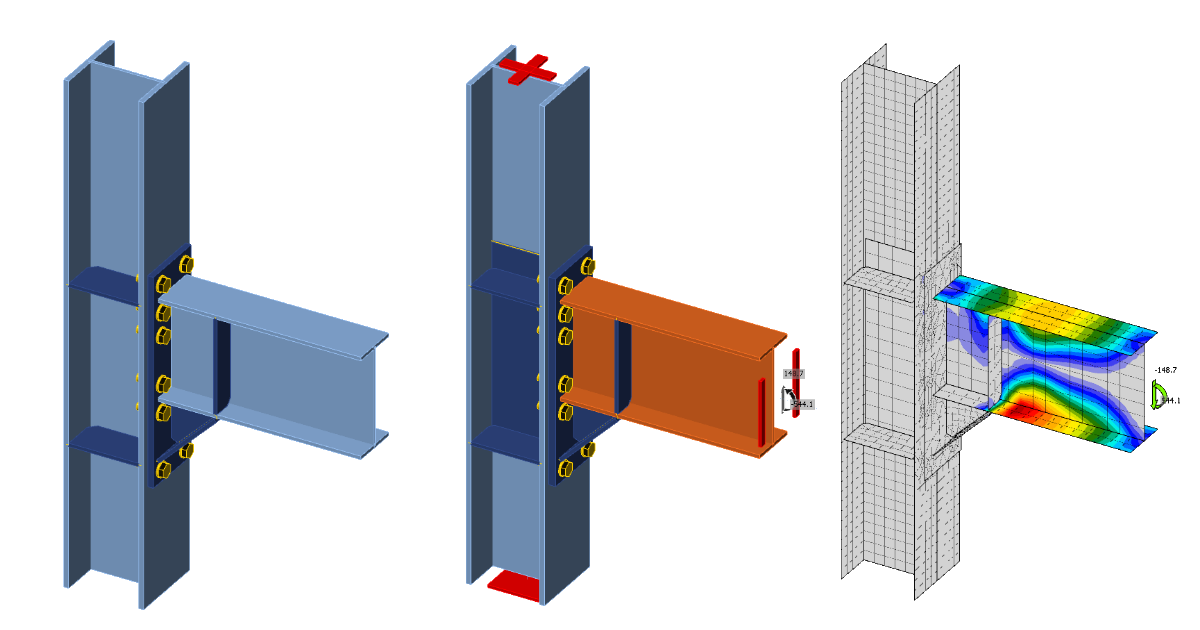# Capacity design (EN)

By following it step-by-step, you will learn how to design and perform seismic code-check of a structural steel joint using Capacity design analysis type based on CBFEM in IDEA StatiCa Connection.
$$1 New projectLaunch IDEA StatiCa (download the newest version) and open the source project file. The joint design is finished, and it is prepared for the standard Stress/Strain analysis.2 Calculation and checkStart the stress/strain analysis by the Calculate button in the ribbon. The analysis model is automatically generated, the calculation is performed, and you can see the overall check results in the left top corner of the scene.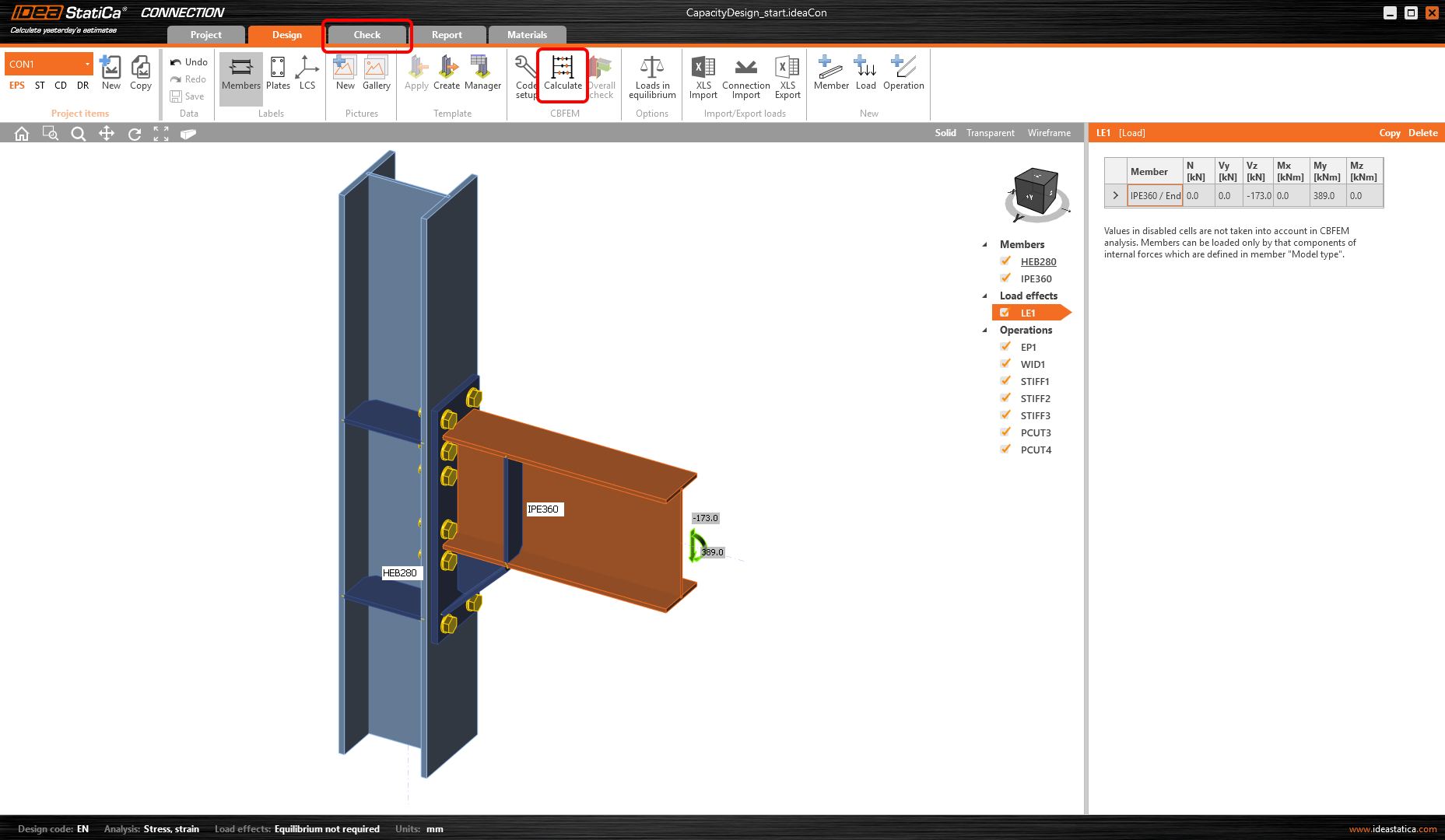You can see that based on the stress/strain analysis, the joint is well designed and passed all the checks.To keep these results, copy this project item.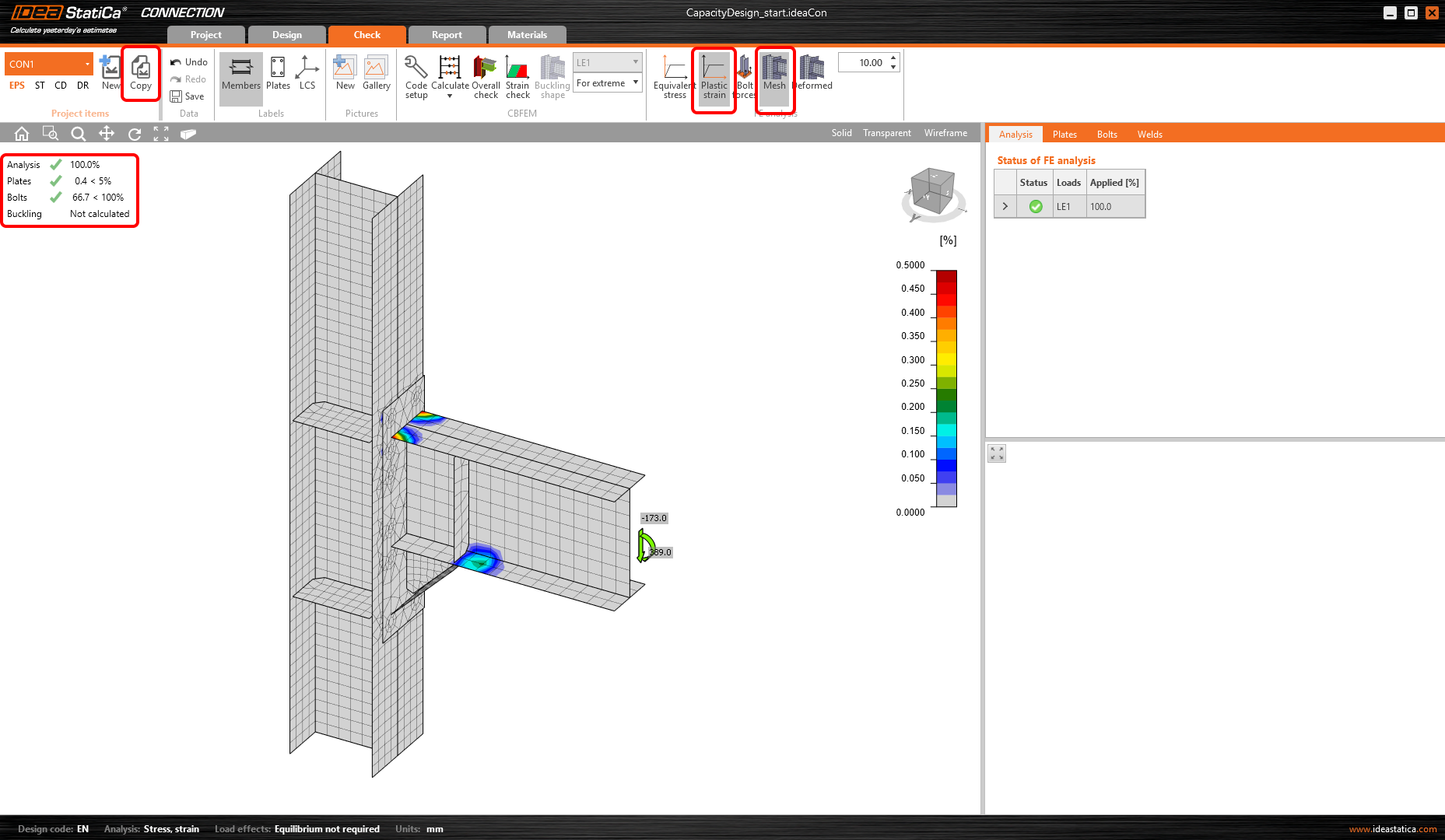3 Capacity checkIn the new project item (CON2), change the analysis type to CD – Capacity design.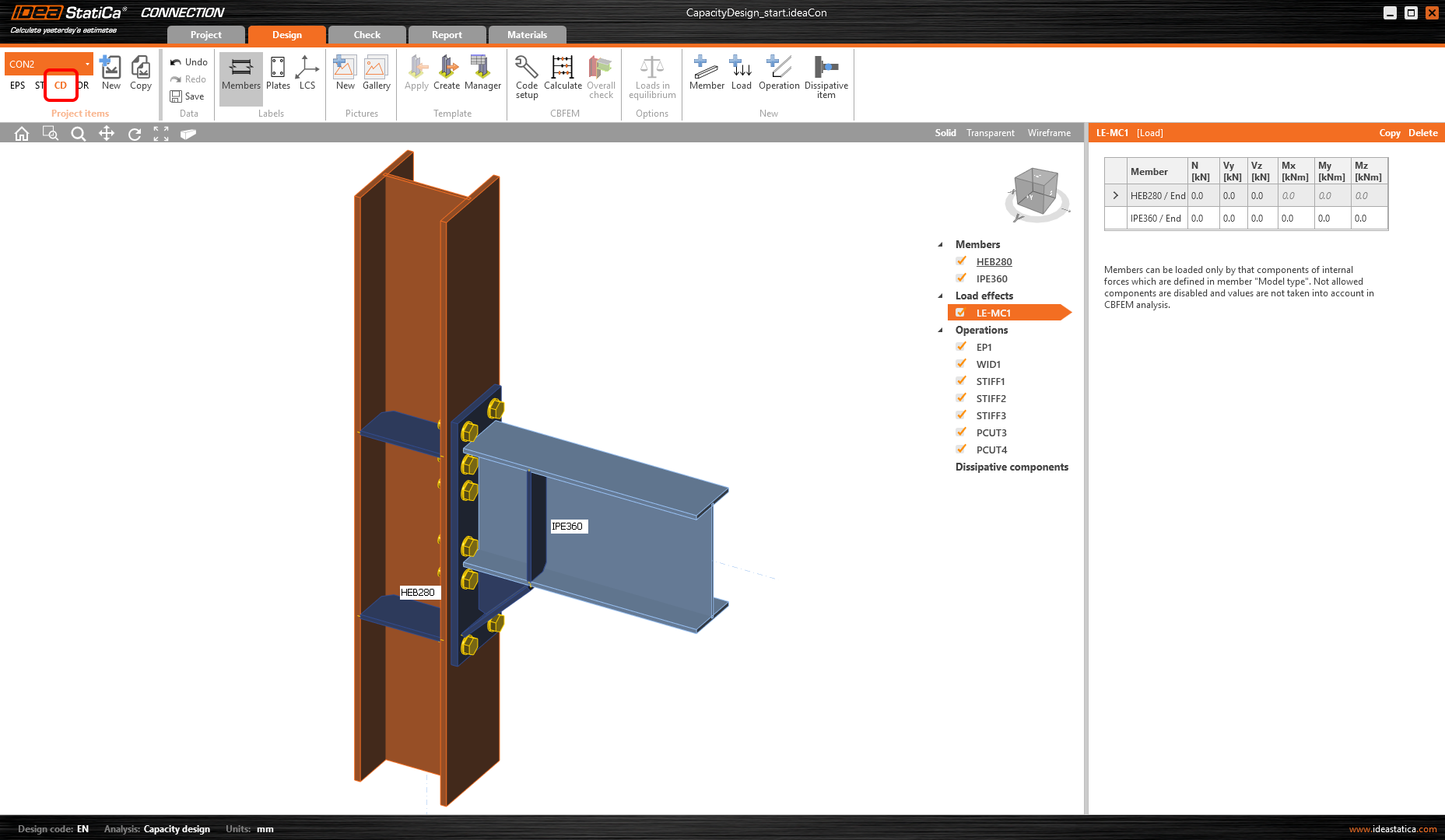The dissipative item has to be selected. It can be added by the command from the top ribbon or by the mouse right-click in the tree in the scene.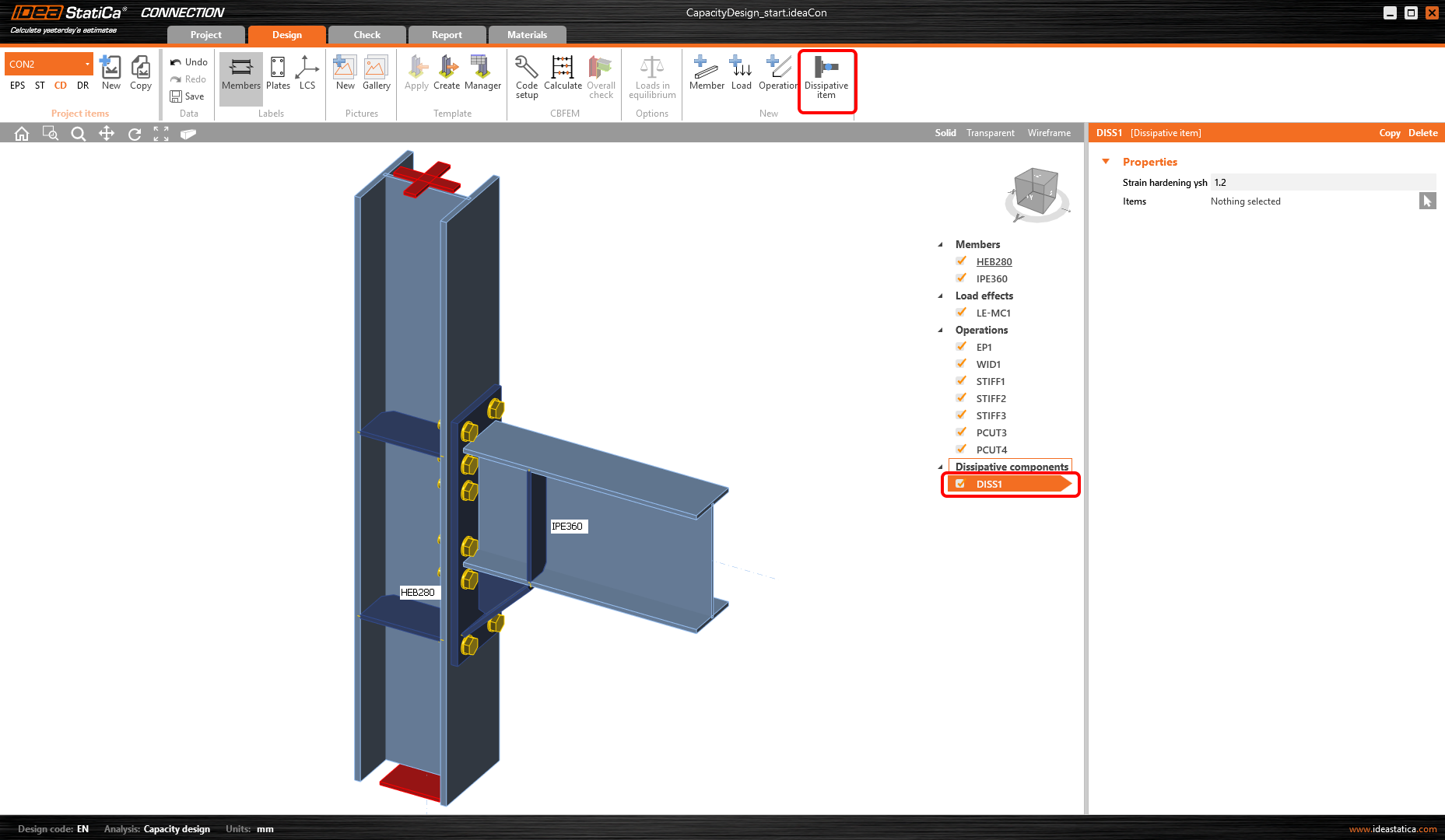A member or a plate where a plastic hinge is expected to occur should be chosen as a dissipative item. The material overstrength factor and strain-hardening factor are applied to the chosen item. In this example, select the member IPE360 as the dissipative item and confirm the selection with the spacebar/enter key/right-click.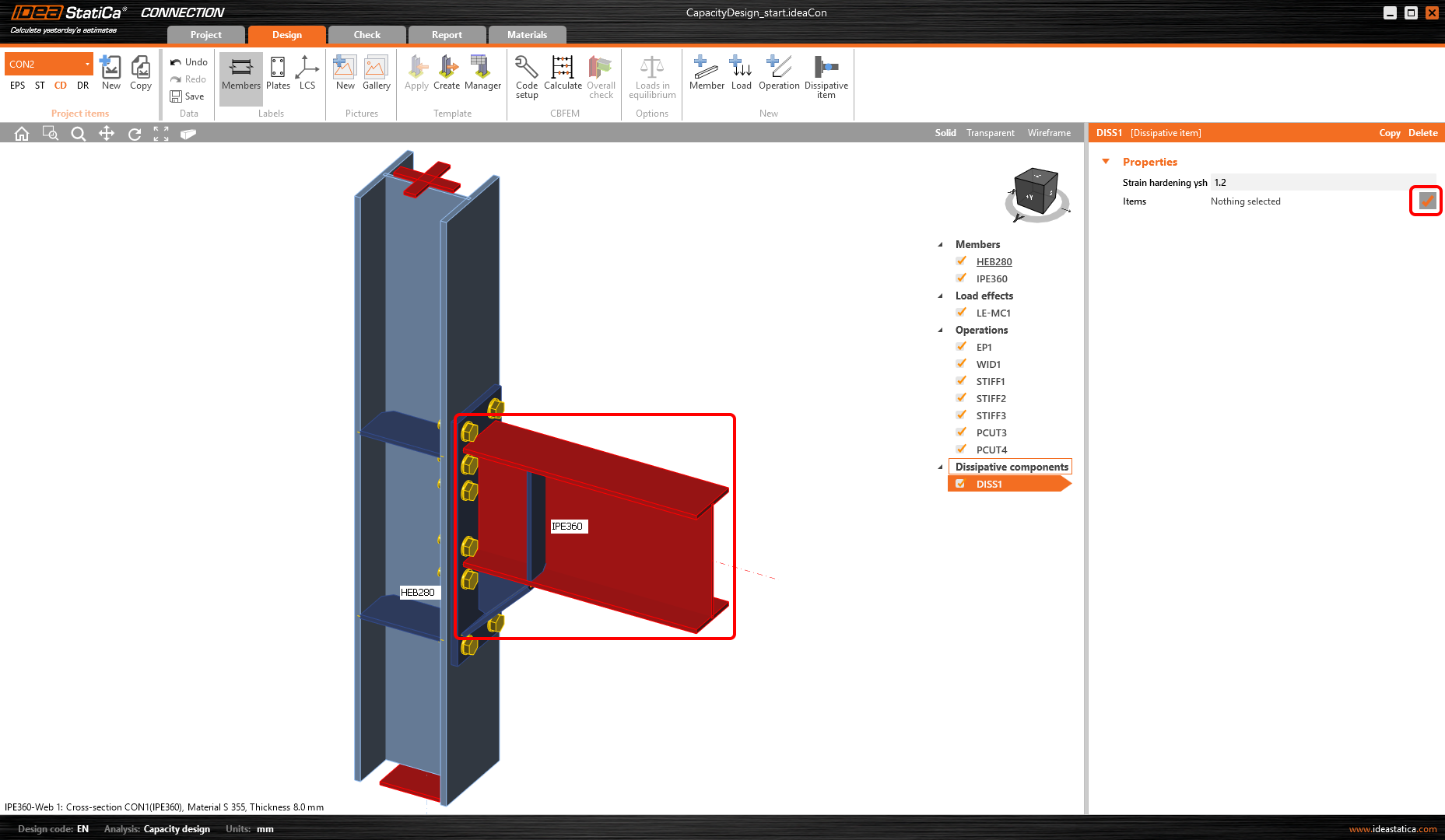In the properties Members, the parameters of IPE360 have to be adjusted: Set the Model type to N-Vz-My, because the connection can resist the bending moment in the vertical plane only and bending around minor beam axis must be restrained. Switch the Forces in parameter to Position, because then the exact acting force position can be defined. The position of the plastic hinge is similar to the position of the acting force: X = 365 mm.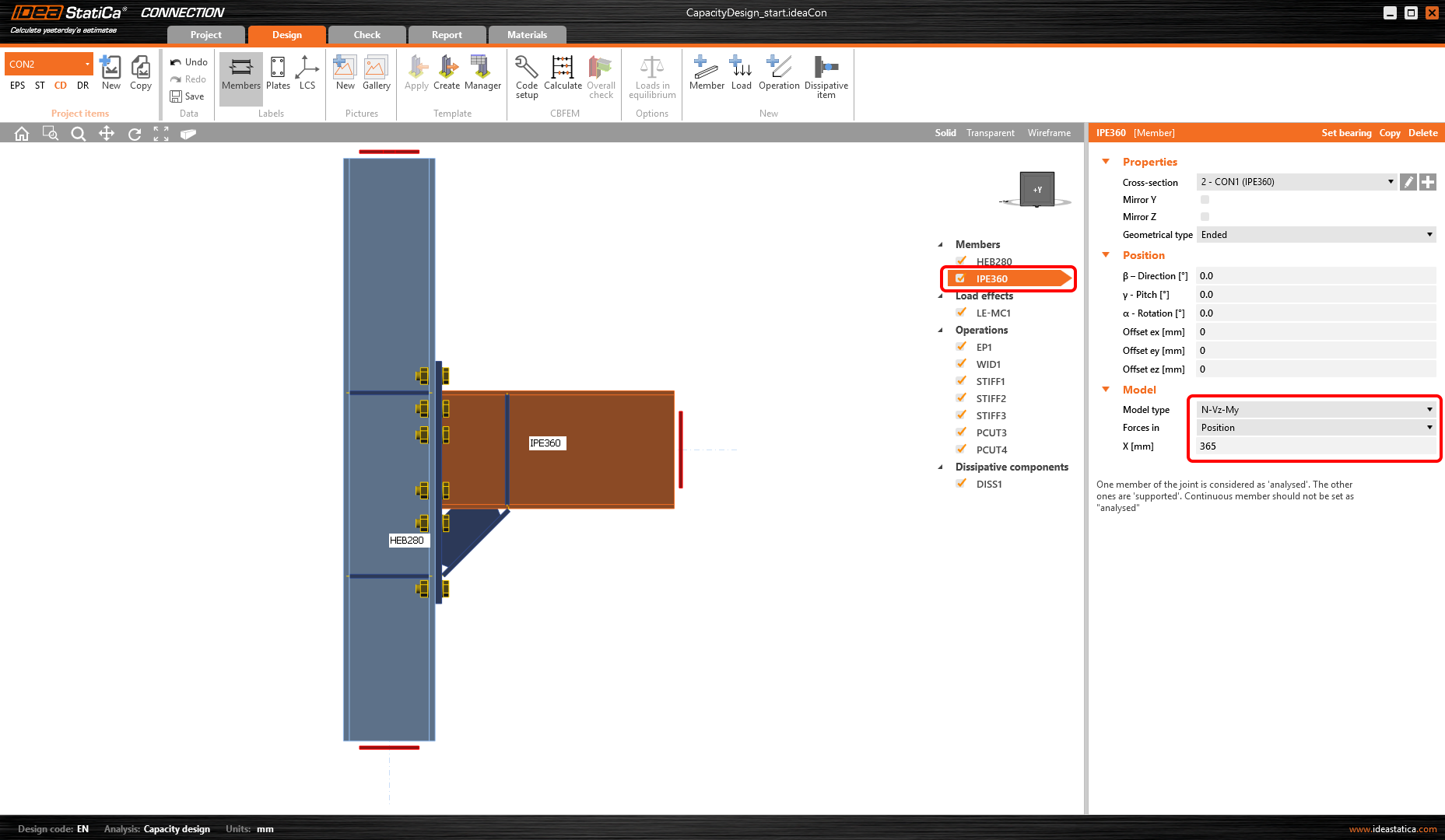How to know the right position of the plastic hinge? The engineer needs to decide where it will occur. Usually, the plastic hinge is determined on the beam. In this example, it will occur just behind the face of the last stiffener. It’s handy to read the position from the application (wireframe view).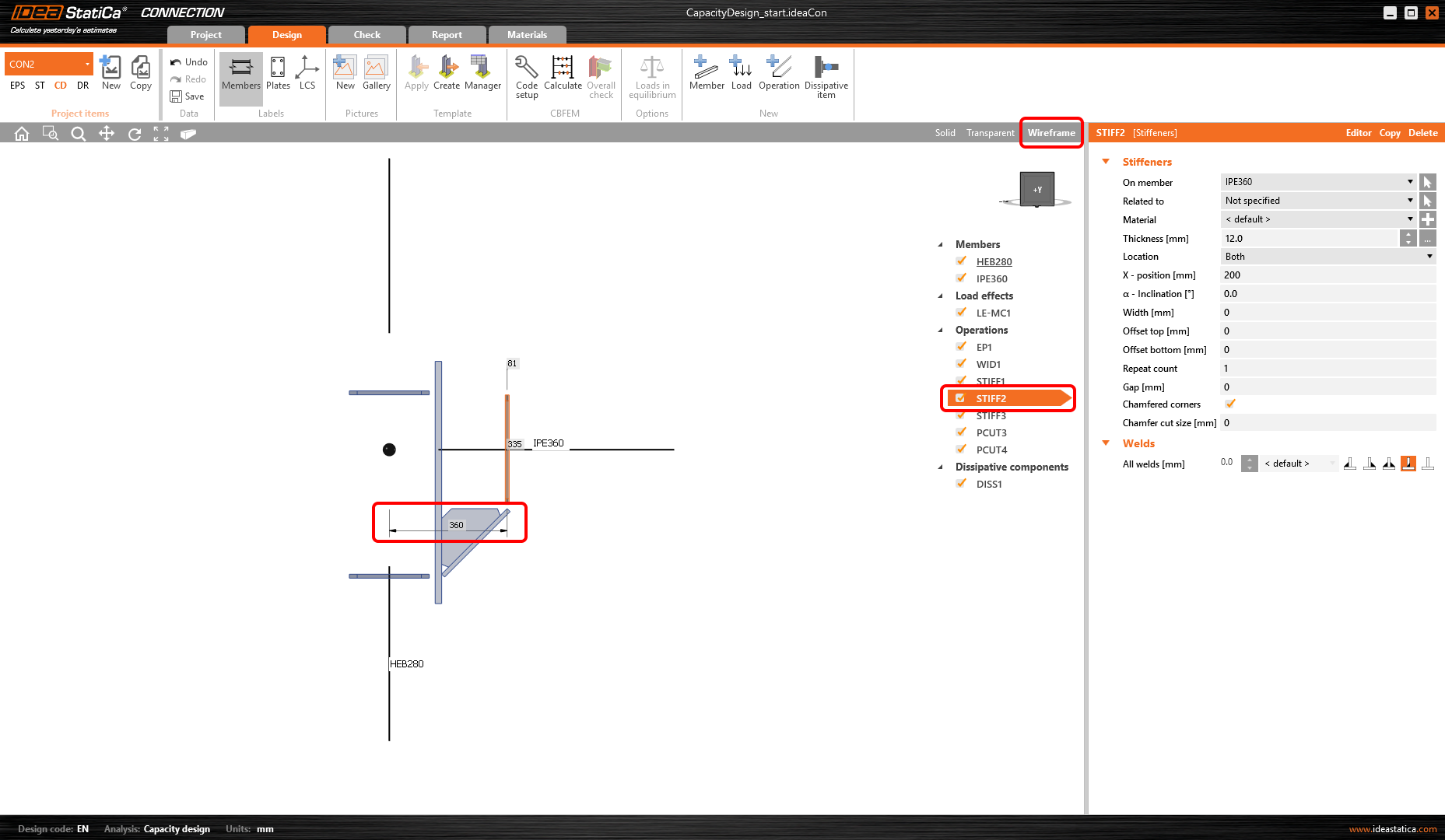In the next step, the load effects have to be defined. Loads for seismic analysis are code dependent (the material overstrength factor, strain-hardening factor) and influenced also by yield strength, geometrical characteristic of the cross-section, etc.Loads for this example were calculated by this procedure:$M_{\textrm{Ed}} = \gamma_{\textrm{sh}} \cdot f_{\textrm{y,ov}} \cdot W_{\textrm{p}l} = 1.2 \cdot 447.75 \cdot 10^6 \cdot 1.0218 \cdot 10^{-3} = 544.12 \, \textrm{kNm}$$$\gamma_{\textrm{sh}} = 1.2$$ $$f_\textrm{y} = 355 \, \textrm{MPa}$$$$f_{\textrm{y,ov}} = f_\textrm{y} \cdot \gamma_{\textrm{ov}} = 355 \cdot 1.25 = 443.75\, \textrm{MPa}$$$$\gamma_{\textrm{ov}} = 1.25$$ $$W_{\textrm{pl,IPE360}} = 1.0218 \cdot 10^6 \, \textrm{mm}^3$$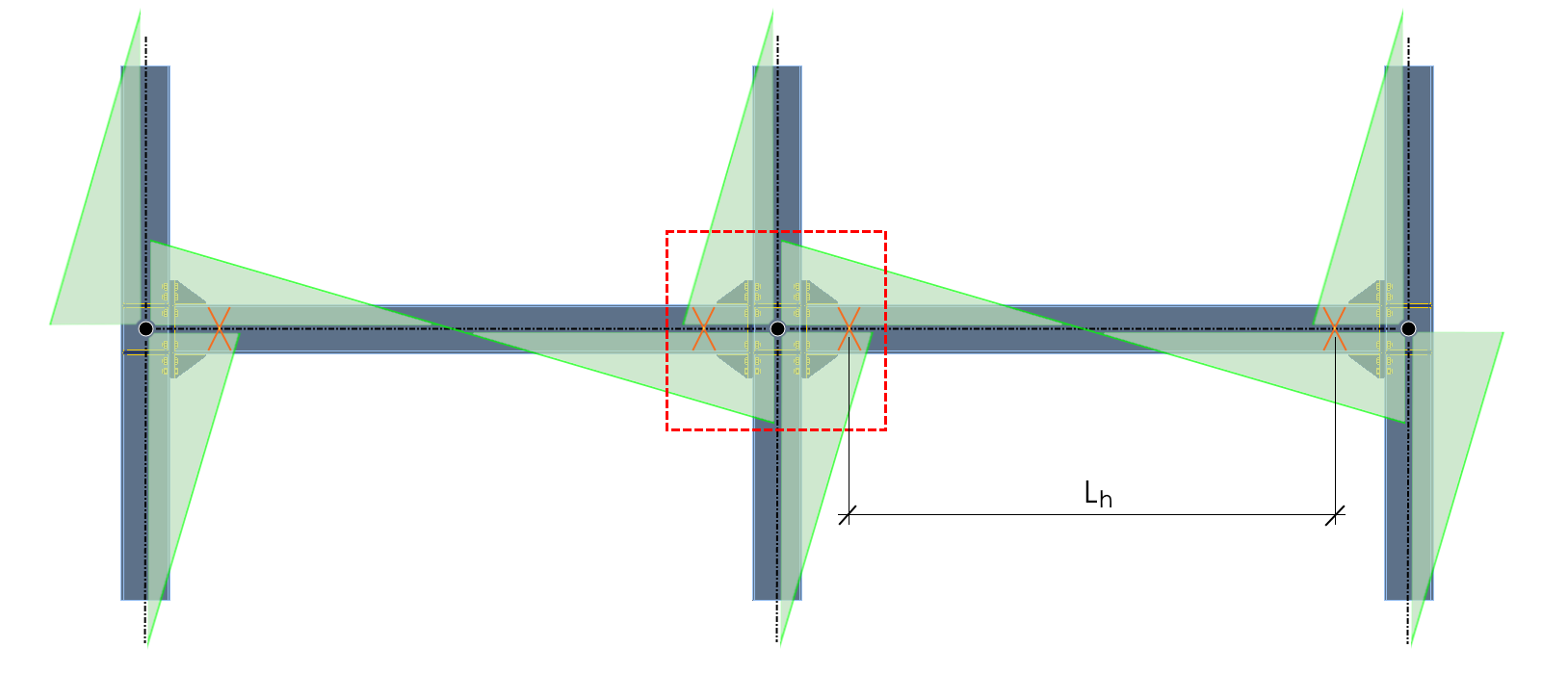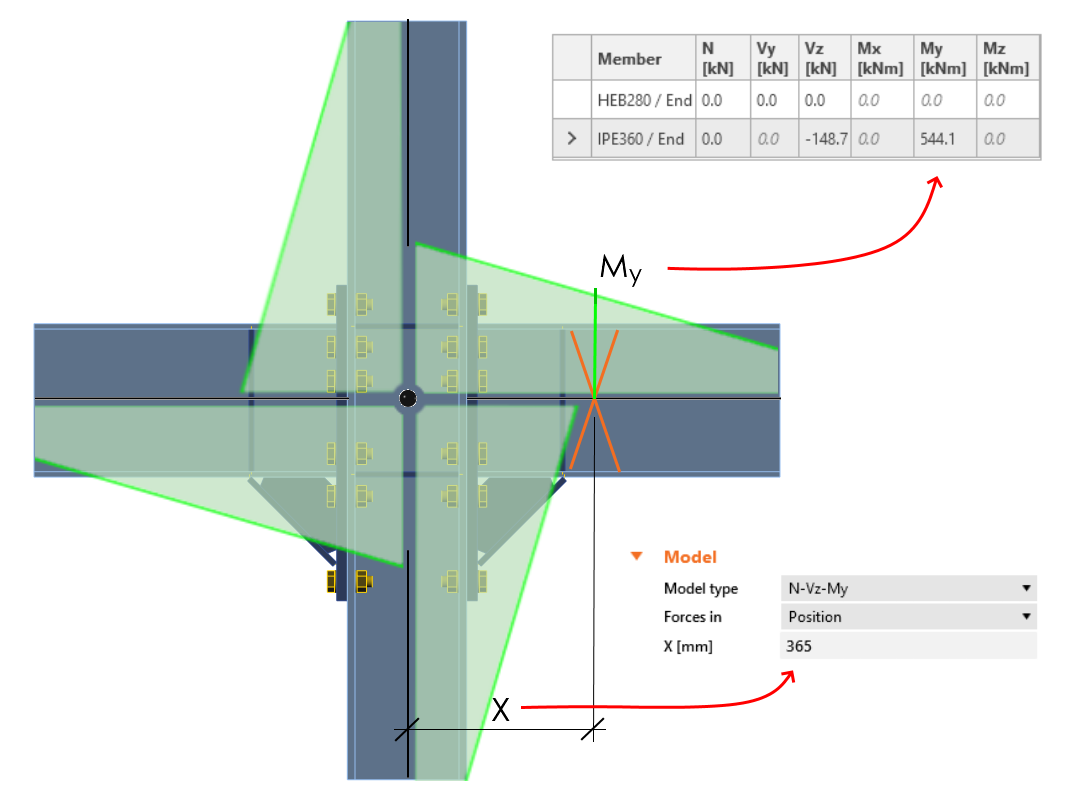$V_{\textrm{Ed}} = \frac{2 \cdot M_{\textrm{Ed}}}{L_{h}} = 2 \cdot \frac{544.12}{7.32} = 148.67 \, \textrm{kN}$$$L_{h} = 7.32 \, \textrm{m} \, -$$ distance between plastic hinges on the beamAdd the calculated shear force and bending moment as a new Load effect (LE).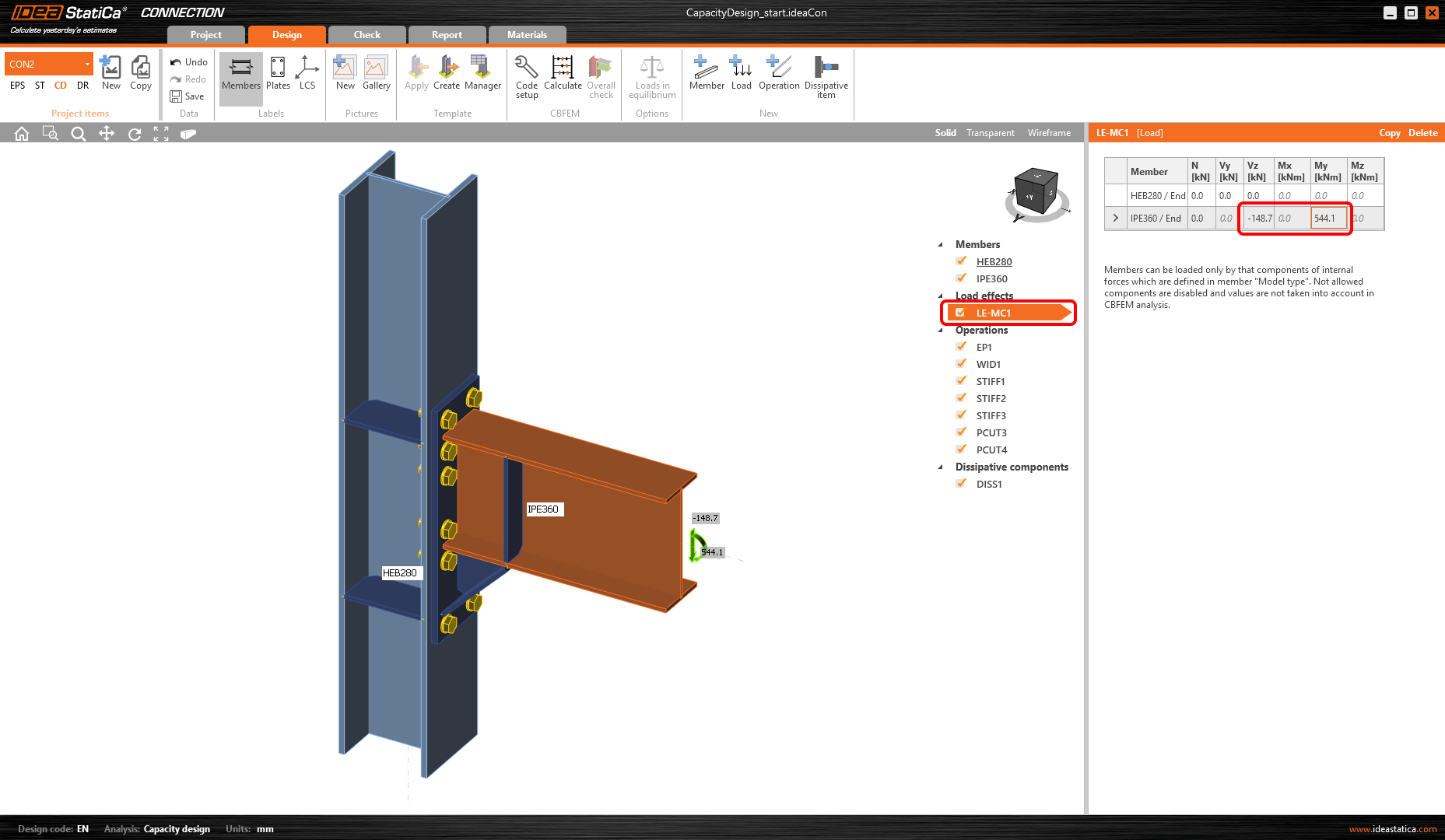The shear force and bending moment must be with appropriate signs so that the bending moment is decreasing on the beam in the direction away from the node.Copy this LE and change the orientation of the acting forces so that the second LE will act in the opposite direction.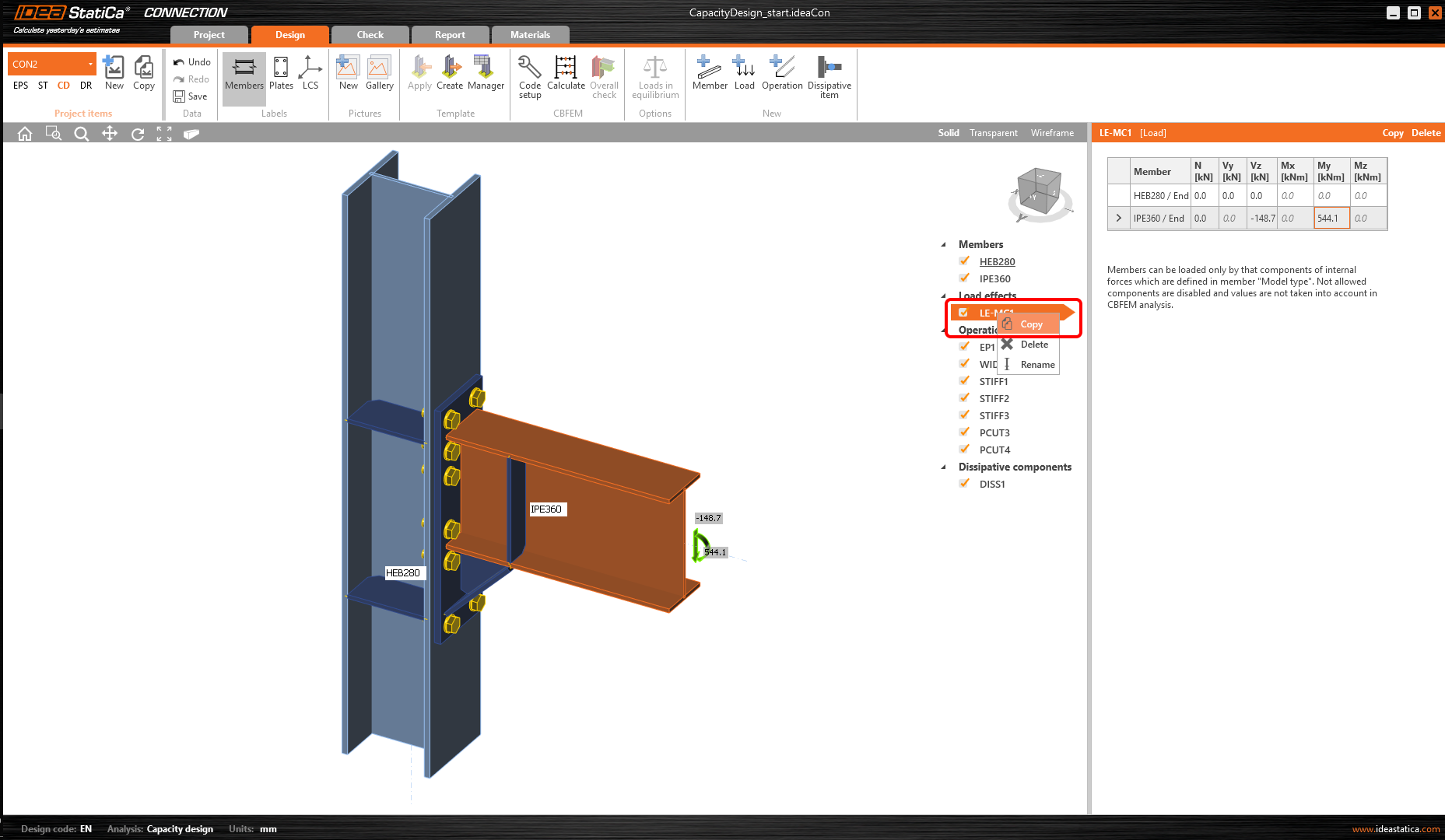Now the capacity analysis can be started by Calculate command.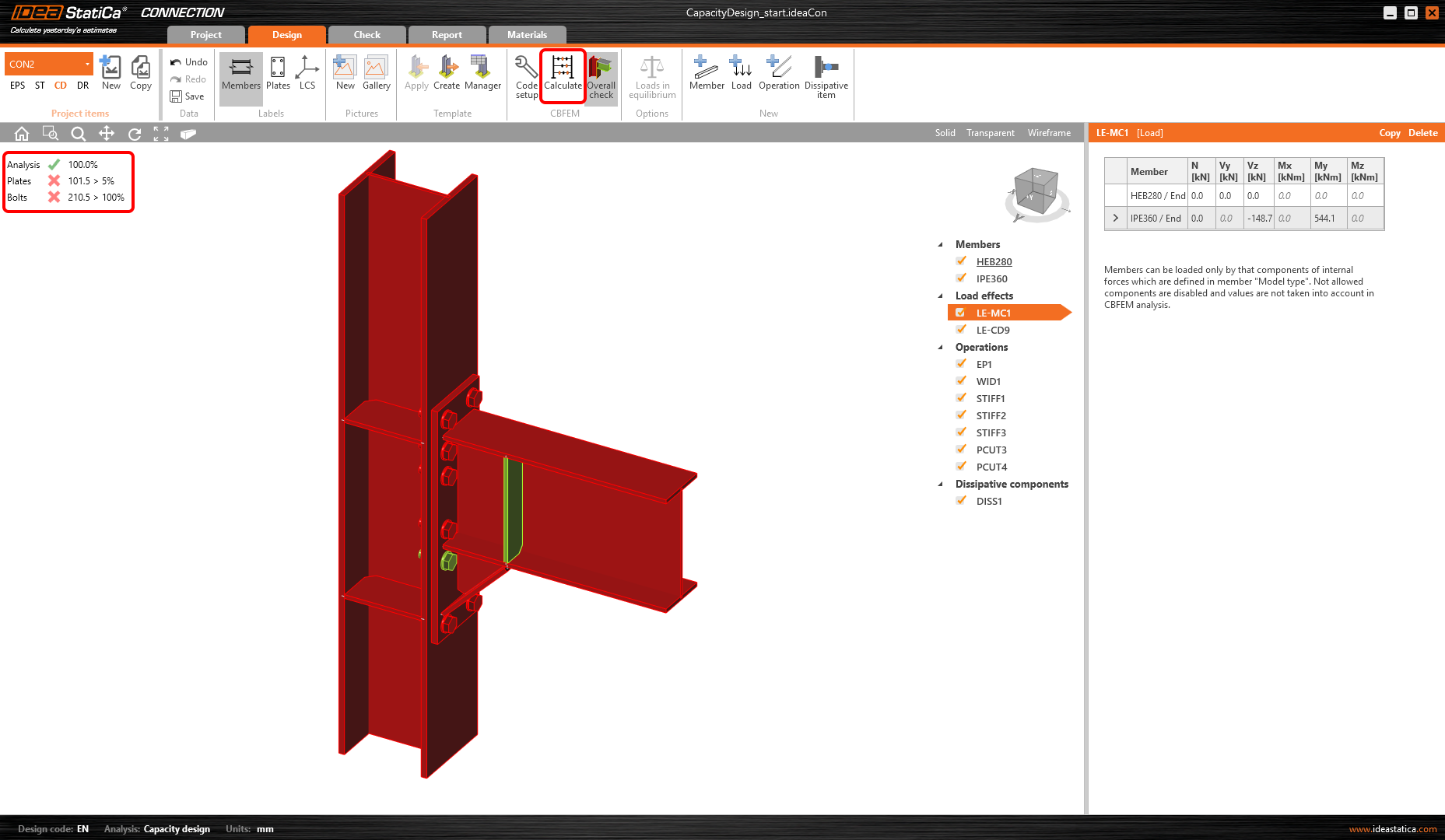You can see from the results that the joint didn’t pass the code check. Some changes in design are required.Increase the thickness of the endplate to 25 mm to prevent it from collapsing.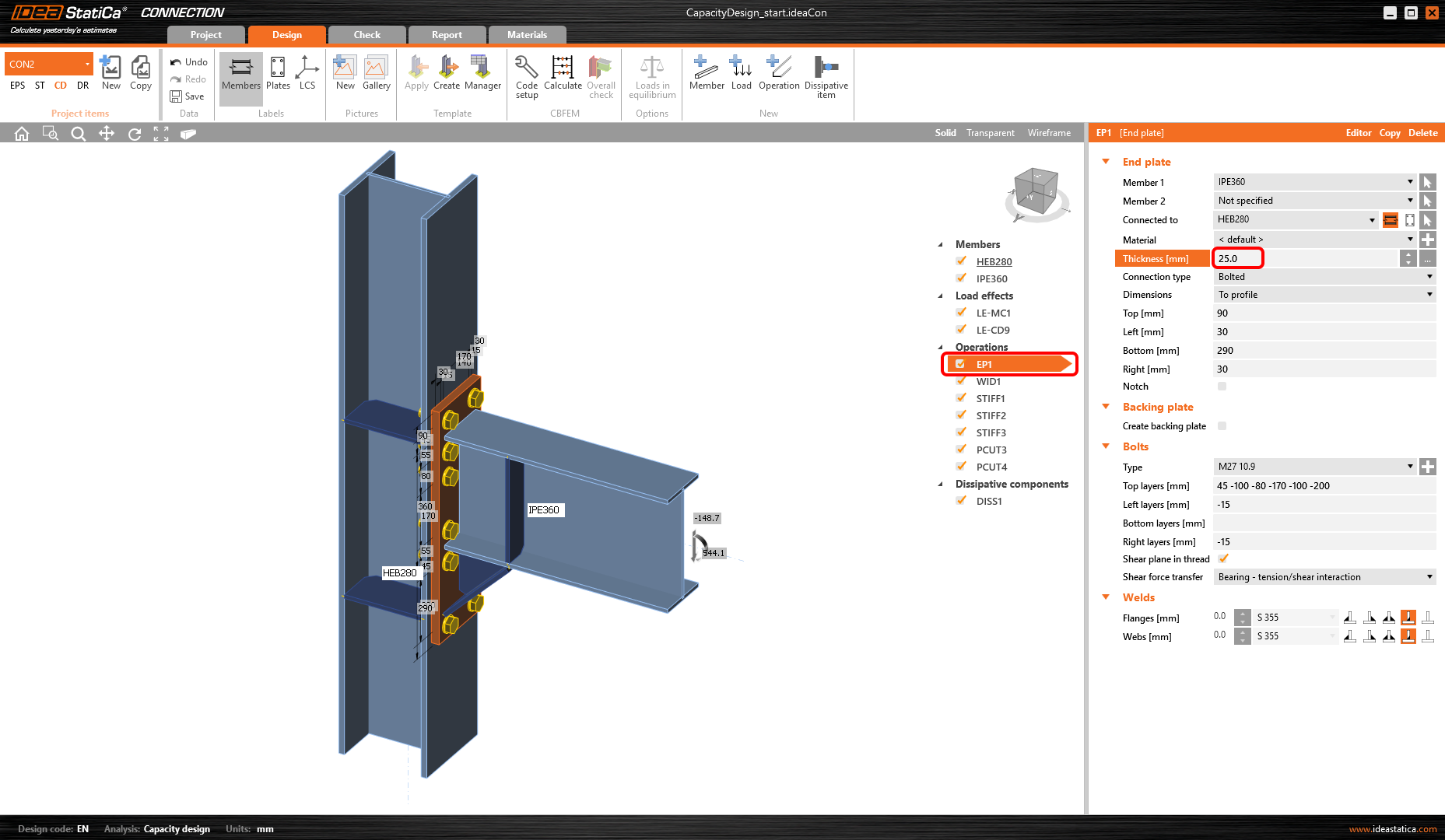To increase column load capacity, add a doubler to its web (add Stiffening plate manufacturing operation).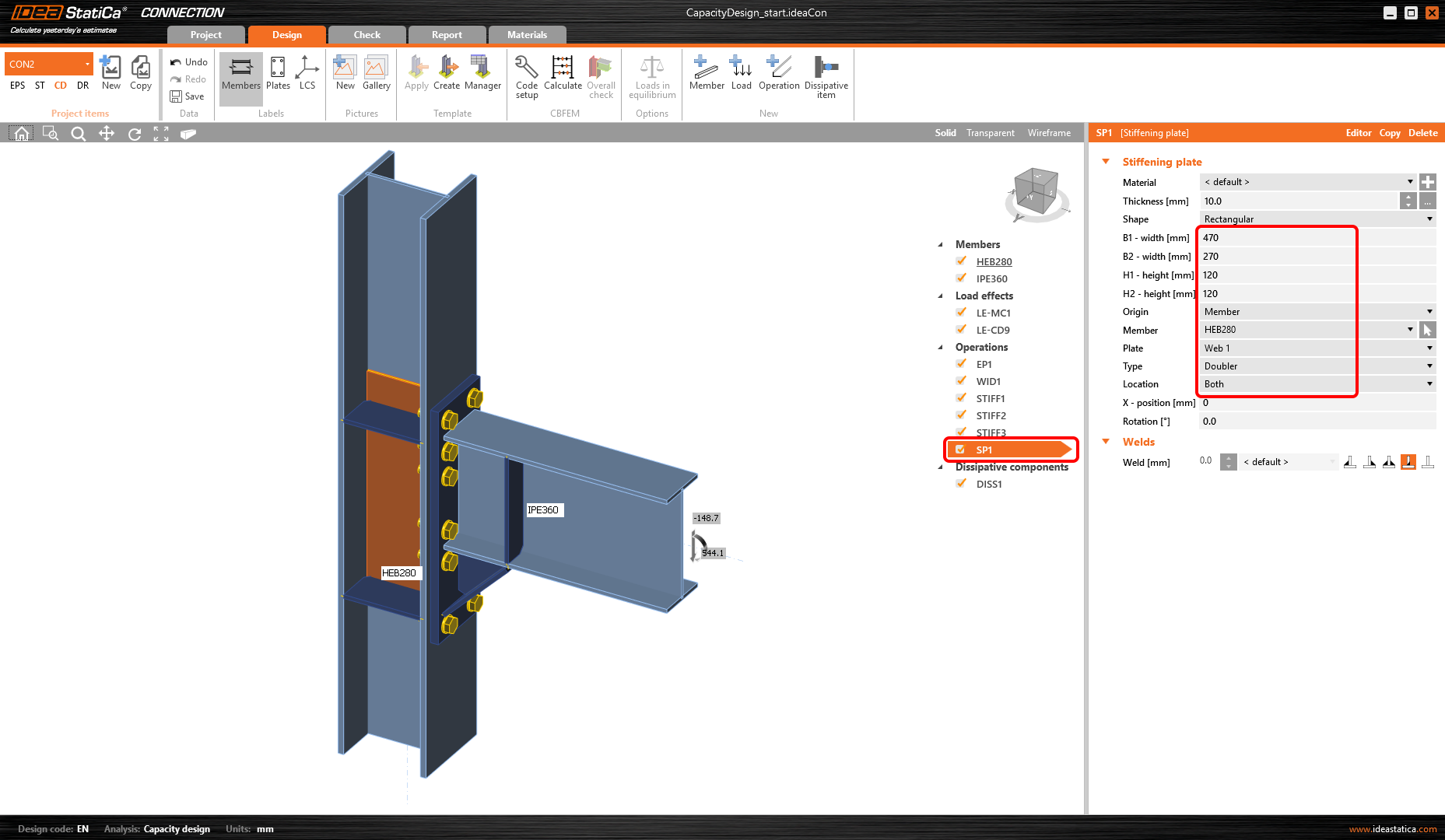The doubler is welded by butt welds to the web of the column, weld to the flanges need to be defined as well.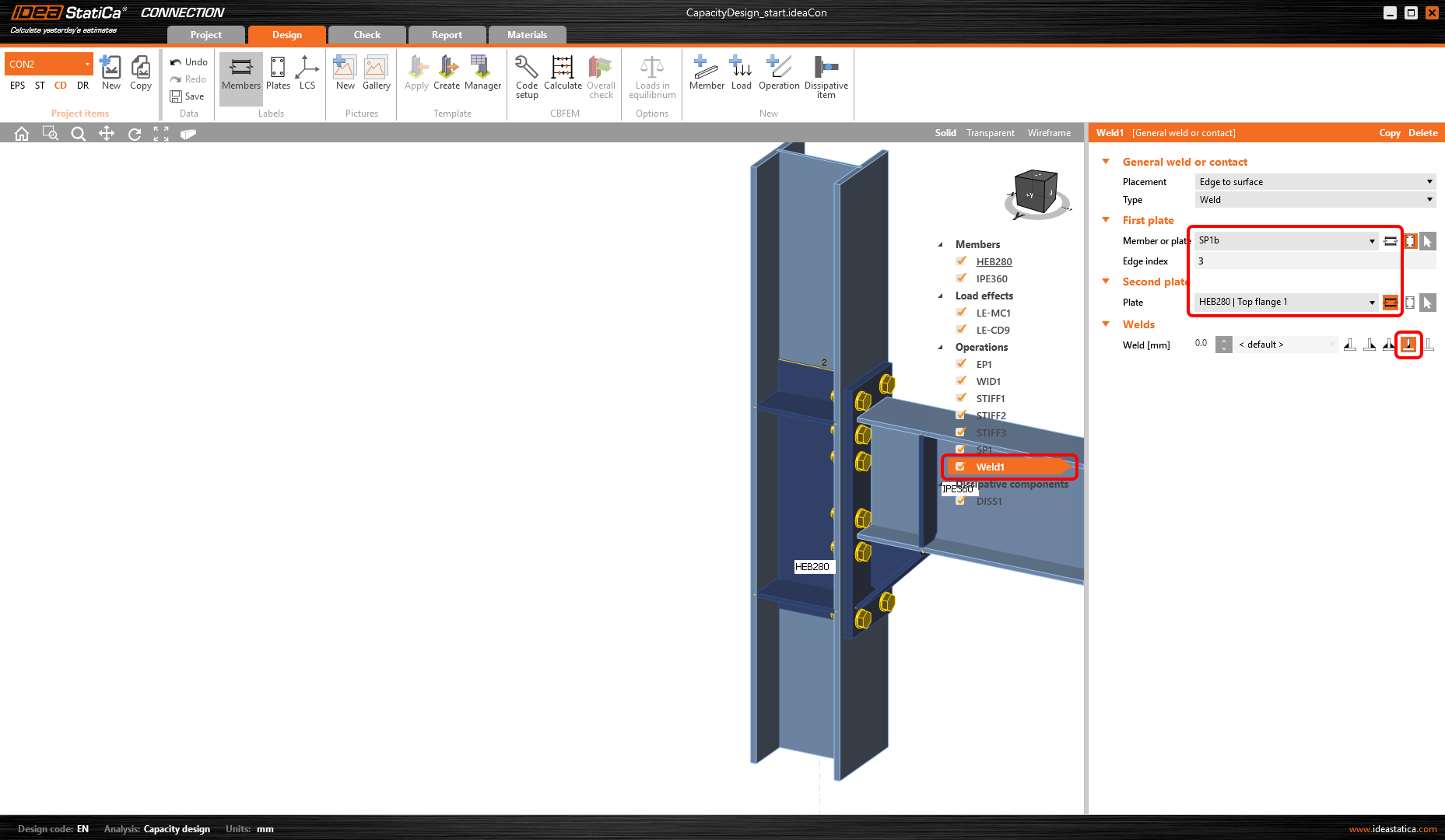Four welds have to be added to weld doublers on both sides of the column to both flanges.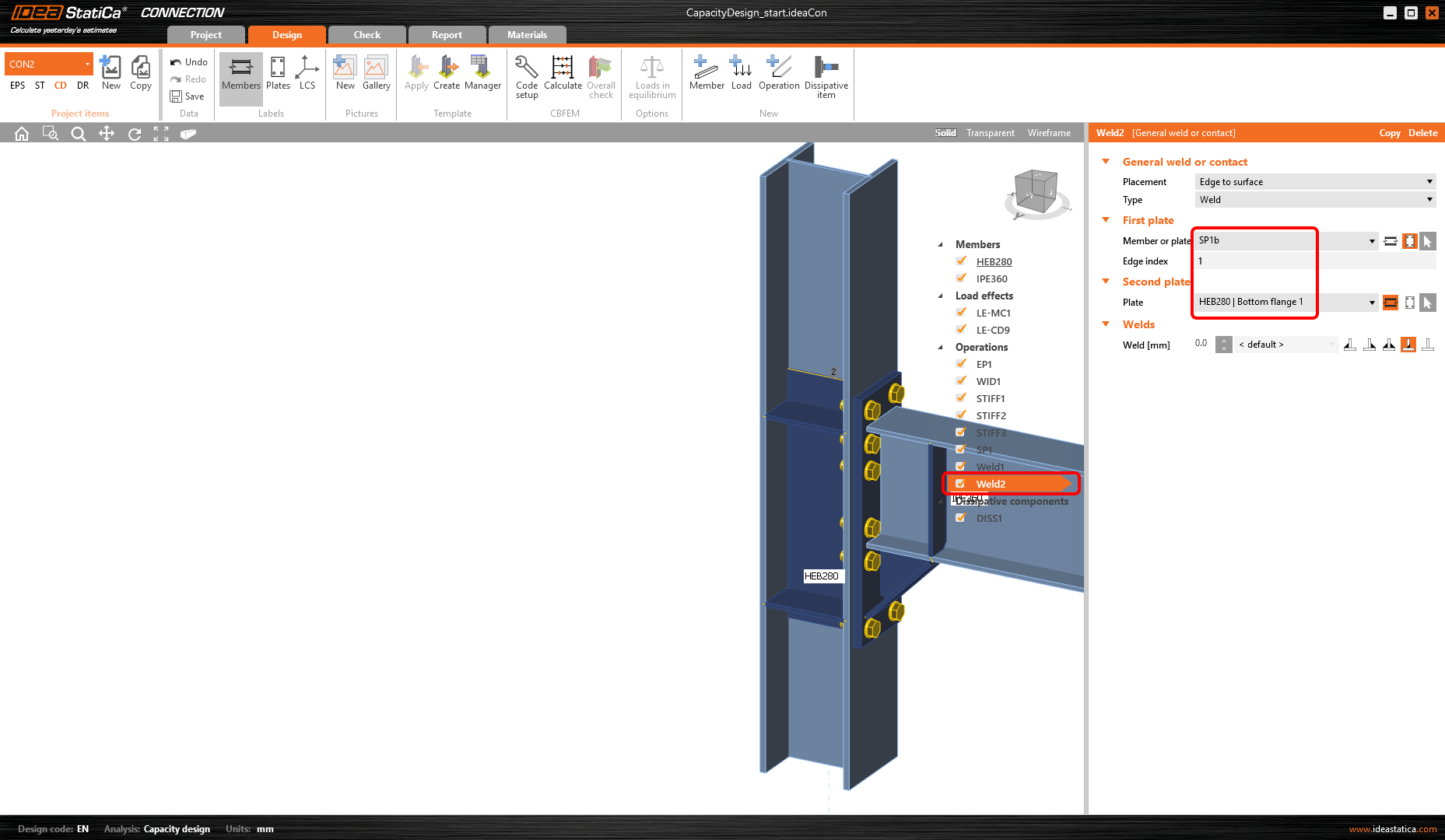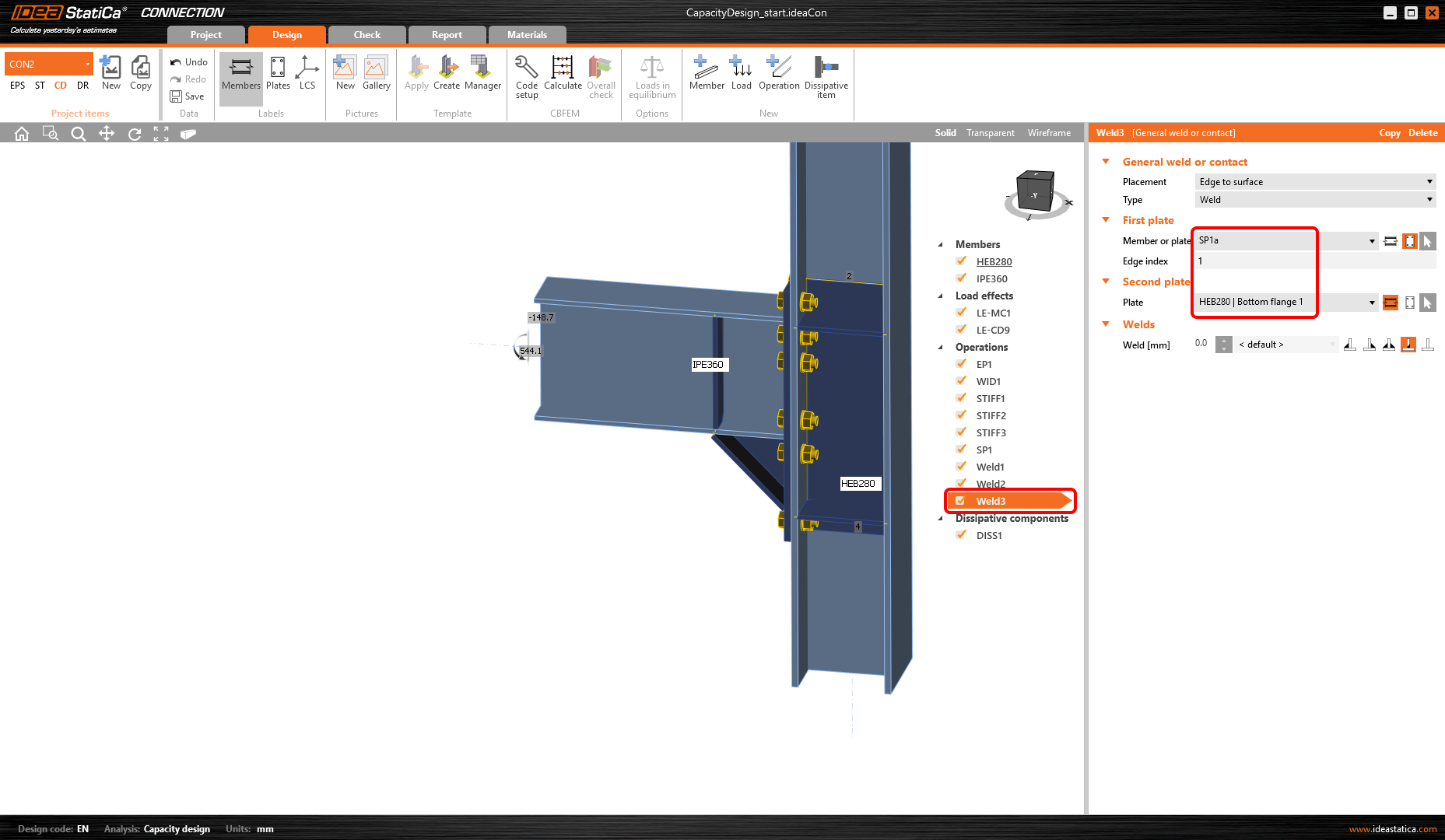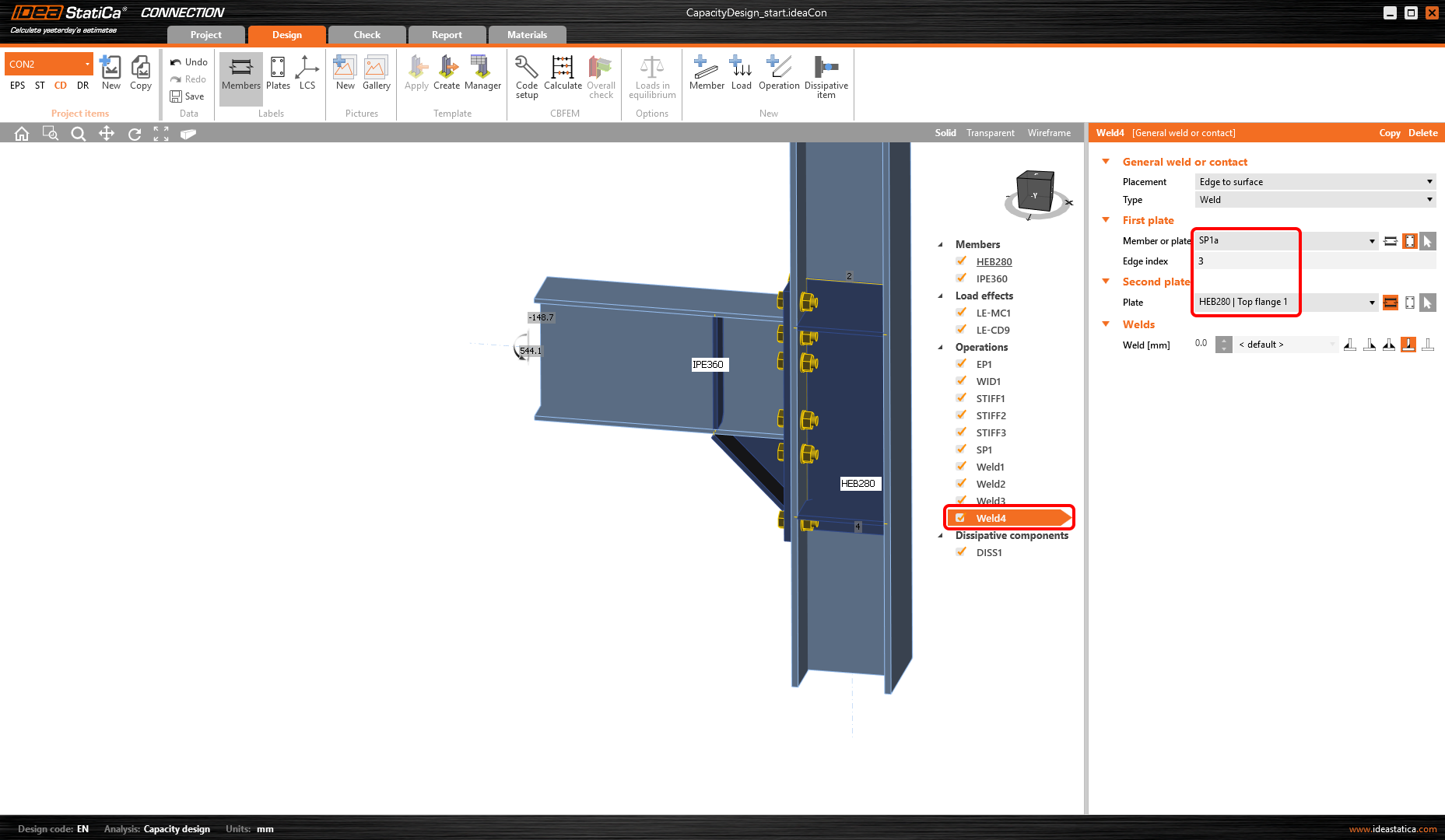The stiffeners at the column web need to be cut and welded to the doublers by the Cut of the plate manufacturing operation.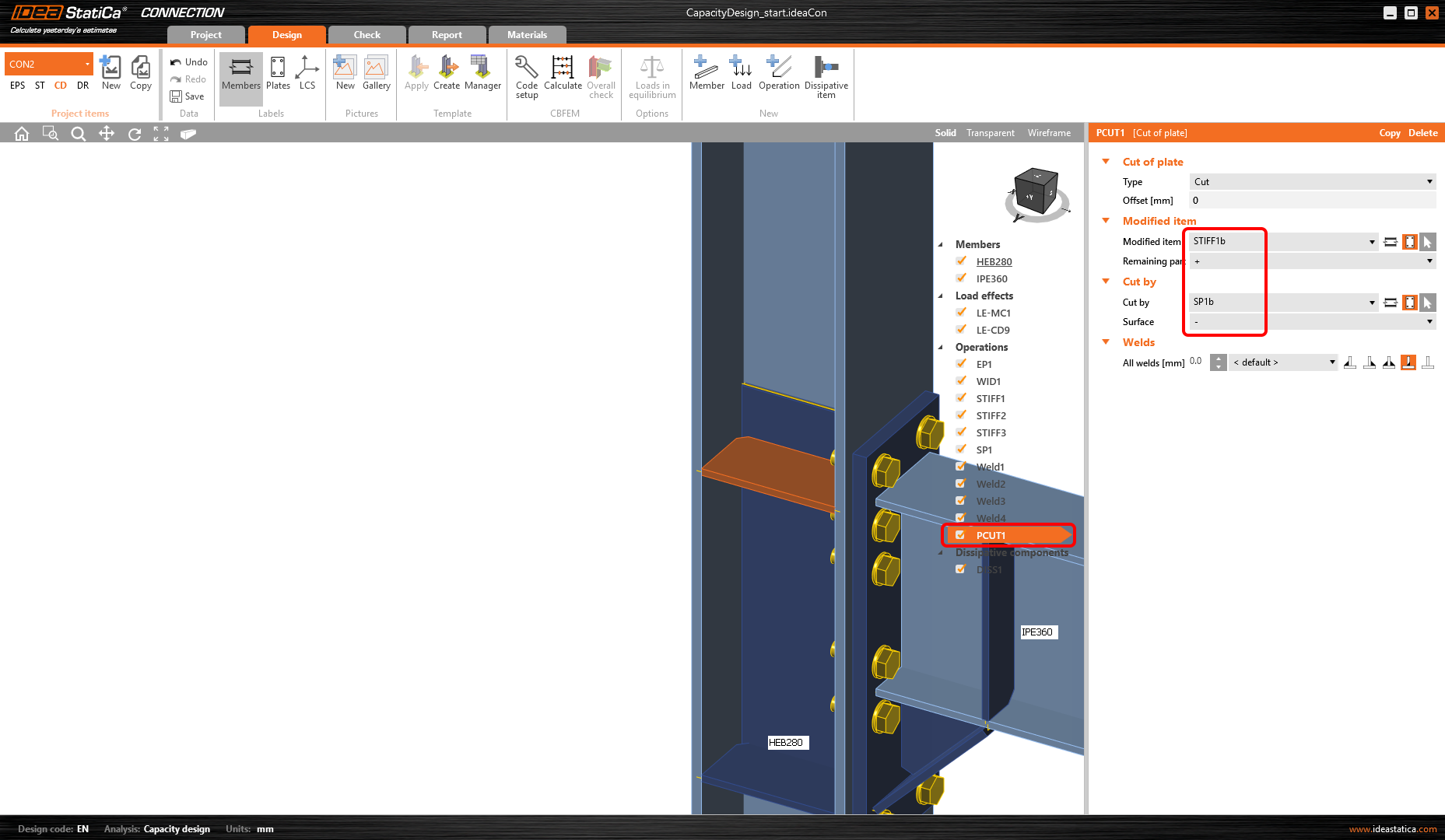Repeat the cut of plate to connect all four stiffeners to the doublers.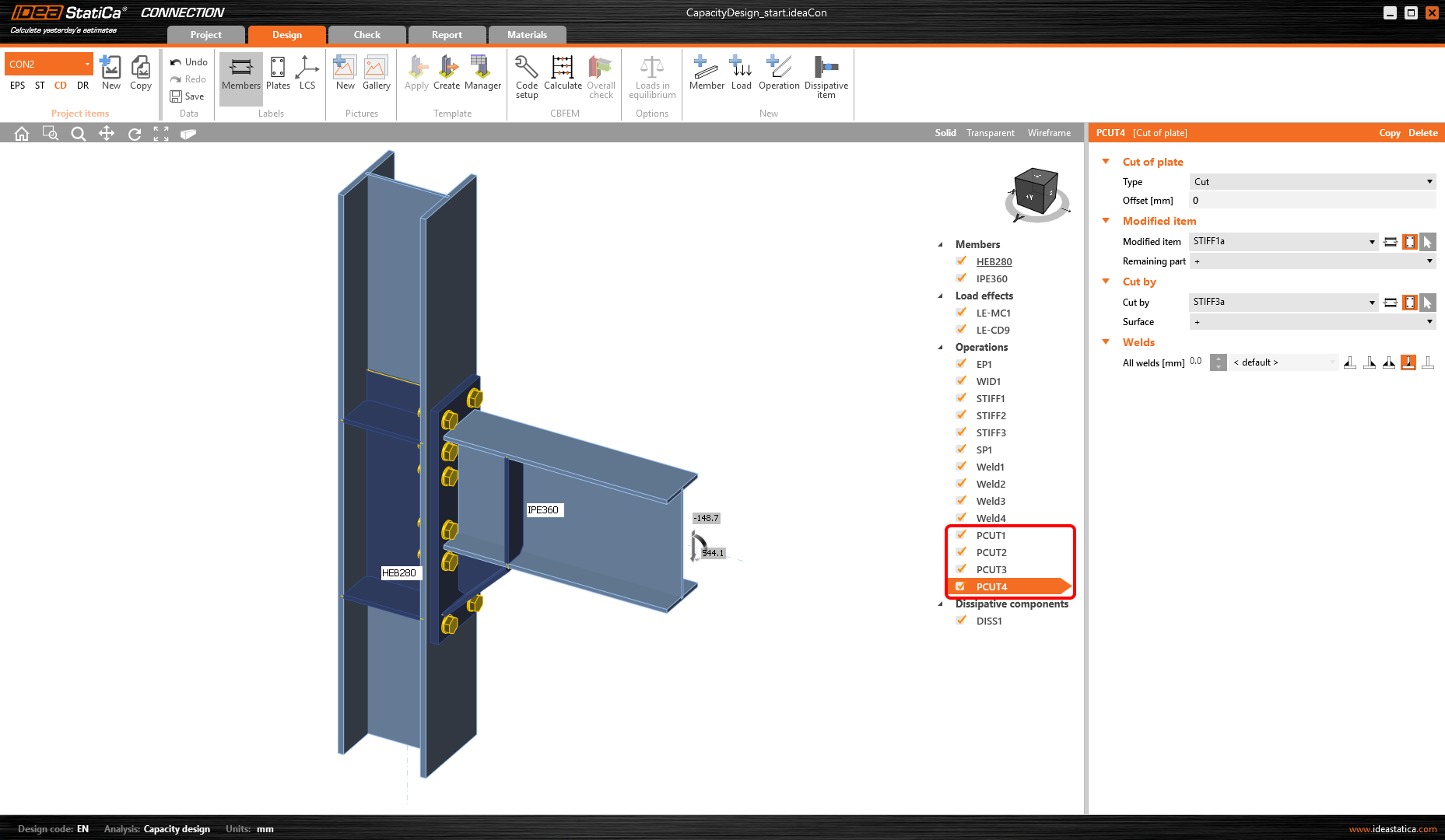All design actions are done now, run Calculate in the Check tab. You can see that all components (like welds and bolts) passed the code-check. The plastic strain of the dissipative item plates does not influence overall results.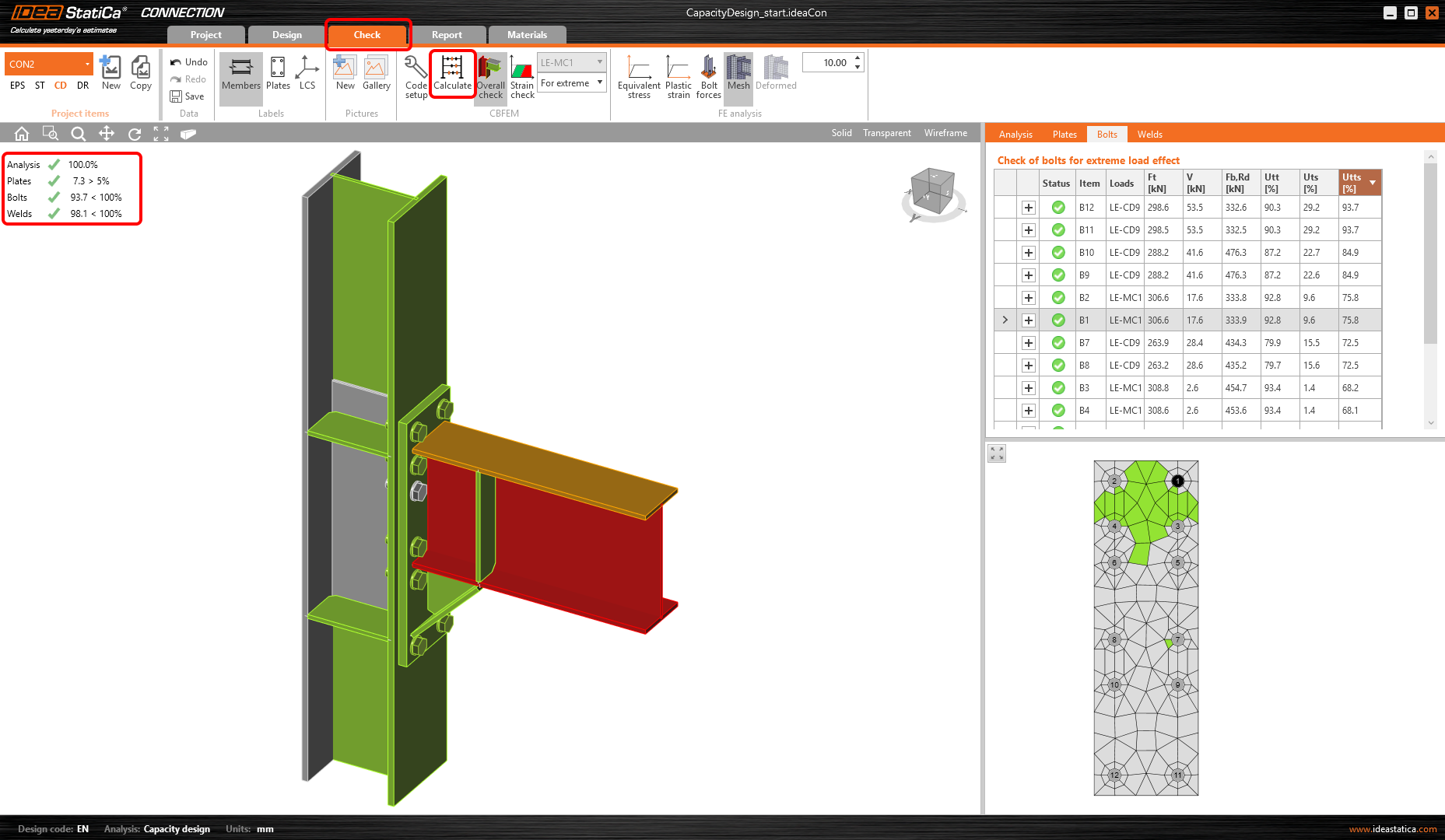The occurrence of the plastic hinge can be explored if the Plastic strain.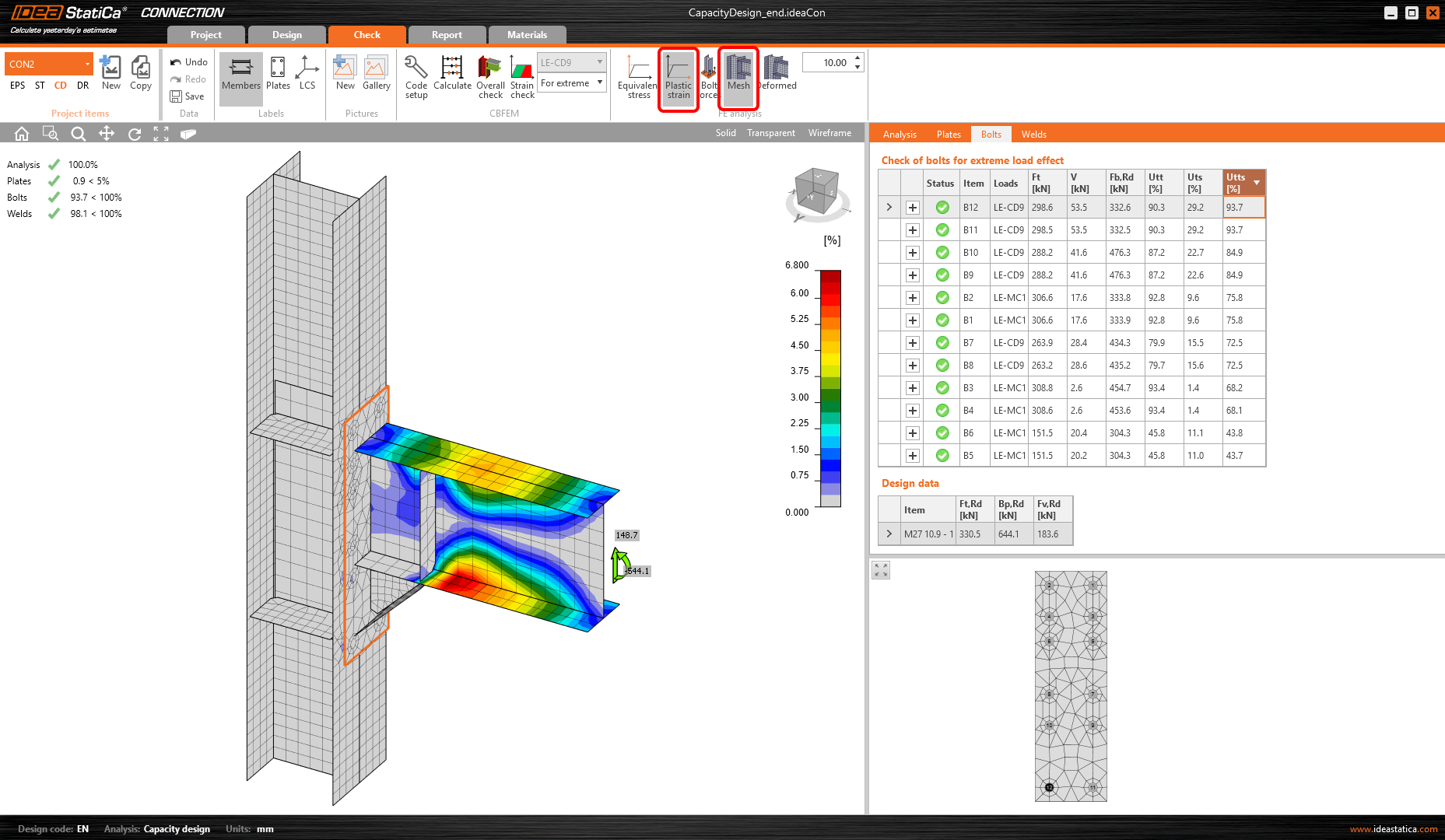The plastic hinge appeared at the expected location and this joint passed the checks required by the capacity design.For a better understanding of the results, see the Theoretical Background.4 ReportAt last, you can review the Report. IDEA StatiCa offers a fully customizable report to print out or save in an editable format.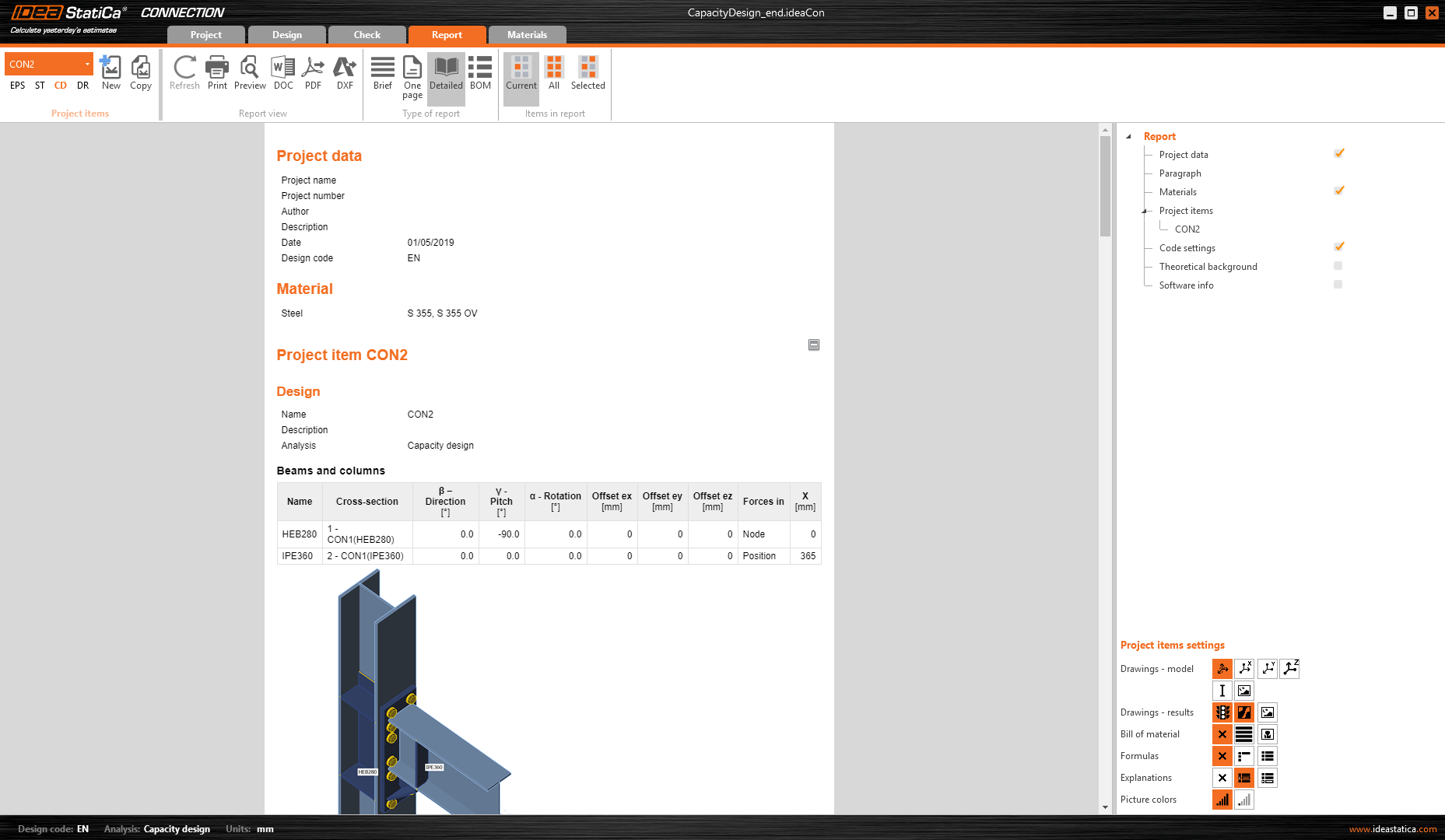You have performed a capacity design check of a structural steel joint according to Eurocode (EN). Sample files Open in Viewer Capacity design (EN)$$